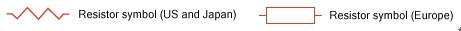# What is a resistor?【Synton-Tech】

## What is a resistor?

What is a resistor?

Resistors are electrical components or electronic components. They are components that have a certain resistance to the flow of current. Resistors can regulate the current and voltage in the circuit.【Symbol of resistor】

Resistor resistance inhibits the flow of current, resulting in a voltage drop (voltage drop). Resistors are widely used in electrical and electronic circuit design.
Resistors can be divided into providing fixed resistors, variable resistors, sliding wire resistors, sliding rheostats, sliding resistors, or adjustable resistors. Adjustable resistors are usually referred to as rheostats, or potentiometers. The resistance value is expressed in ohms and the symbol is Ω.
In electrical or electronic circuit design, resistors can construct a known voltage drop or current-voltage relationship. If the current in the circuit is known (the current is measured in amperes A), then the resistor can be used to create a known potential difference (voltage difference), which is proportional to the current. Conversely, in a circuit, if the voltage drop (potential difference) between two nodes is known, then the resistor can be used to create a known current, which is proportional to the potential difference.
Attenuator is a network resistance composed of two or more resistors, also known as a voltage divider.
A line terminator is a special resistor package or block, which is often used to ensure the stability of the overall circuit signal.
The terminal resistance acts like a filter, which can eliminate the electrical noise generated by numerous cables and equipment.